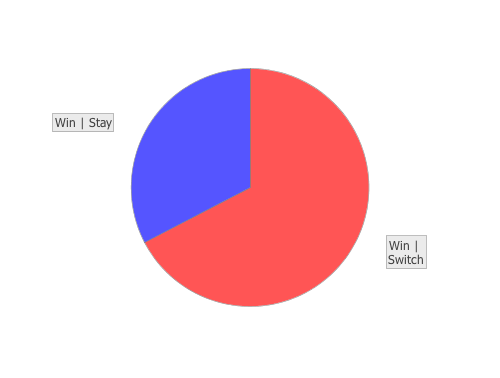# Category Archives: monte carlo simulation

## Monty Hall in Monte Carlo

The Monty Hall problem, named after the host of the Let’s Make a Deal game show, first appeared in Marilyn vos Savant‘s column in Parade magazine in 1990, although people still vigorously debate the answer, as discussed here and here.

The basic premise is that you must choose one of three doors, one of which contains a prize. Once you have selected a door, Monty Hall reveals the contents of one of the doors you did not select, usually containing something like a goat. He then asks if you would like to switch your choice to the remaining unopened door. The question is, will switching doors increase you chances of winning, decrease your chances, or have no effect at all?

One thing to keep in mind, is that when Monty Hall shows you what’s behind one of the doors you didn’t select, he doesn’t ever reveal the door containing the prize. This fact should change your decision making process.

We can answer the question of which strategy is best by calculating the conditional probabilities of winning given you either switch or stay by performing a Monte Carlo simulation. The conditional probability of winning given you decide to switch doors can be written as `Prob(win | switch)`, and is equal to the probability that you switch and win divided by the probability that you switch,

``Prob(win | switch) = Prob(switch & win)/Prob(switch)``

and the conditional probability of winning given you decide to stay with your original door is equal to the probability you stay and win divided by the probability that you stay.

``Prob(win | stay) = Prob(stay & win)/Prob(stay)``

In this simulation, we will generate a bunch of initial guesses, a bunch of prize locations, and a bunch of switch decisions and then use them to calculate the probabilities. First, load the necessary libraries.

``(use '(incanter core stats charts))``

Set a simulation sample size, generate samples of initial-guesses, prize-doors, and switch decisions with the sample function. The probability that the prize is behind any of the three doors is 1/3, as is the probability that you select a particular door as an initial guess, while the probability that you switch doors is 1/2.

``````(def n 10000)
(def initial-guesses (sample [1 2 3] :size n))
(def prize-doors (sample [1 2 3] :size n))
(def switches (sample [true false] :size n))``````

Define a function that returns one if a switch decision results in winning, a zero otherwise.

``````(defn switch-win? [initial-guess switch prize-door]
(if (and switch (not= initial-guess prize-door)) 1 0))``````

Calculate the joint probability of winning and switching, by mapping the switch-win? function over the generated data, summing the occurrences of winning after switching, and then dividing this number by the total number of samples, n.

``````(def prob-switch-win (/ (sum (map switch-win?
initial-guesses
switches
prize-doors))
n))``````

Calculate the probability of switching doors. Use the indicator and sum functions to calculate the frequency of switches, and then divide by the total number of samples.

``(def prob-switch (/ (sum (indicator true? switches)) n))``

Finally, use the formula above to calculate the conditional probability of winning given a switch.

``(def prob-win-given-switch (/ prob-switch-win prob-switch))``

Now repeat the process to calculate the conditional probability of winning given the decision to stay with your original choice. First, define a function that returns a one if a stay decision results in winning, zero otherwise.

``````(defn stay-win? [initial-guess switch prize-door]
(if (and (not switch) (= initial-guess prize-door)) 1 0))``````

Next, calculate the joint probability of winning and staying,

``````(def prob-stay-win (/ (sum (map stay-win?
initial-guesses
switches
prize-doors))
n))``````

and, calculate the probability of staying with the original door.

``(def prob-stay (/ (sum (indicator false? switches)) n))``

Finally, calculate the conditional probability of winning given you stay with the original door

``(def prob-win-given-stay (/ prob-stay-win prob-stay))``

View the results with the pie-chart function.

``````(view (pie-chart ["Switch" "Stay"]
[prob-win-given-switch prob-win-given-stay]))``````The conditional probability of winning given you switch doors is approximately 0.66, while the probability of winning given you stay with your original choice is approximately 0.33.

To some this outcome may seem non-intuitive. Here’s why it’s the case: the probability that the prize is behind any of the three doors is 1/3, so once you have selected a door, the probability that the prize is behind a door you didn’t select is 2/3. Since Monty Hall knows which door has the prize, and never selects it as the door to reveal, all of the 2/3 probability belongs to the other unselected door. So switching will give you a 2/3 probability of winning the prize, and sticking with your original decision only gives you the original 1/3 chance.

The complete code for this example can be found here.